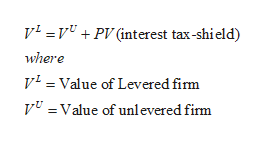# This question is related to Chapter 18 of Berk & Demarzo "Capital Budgeting and Valuation with Leverage".How do the calculations of the firm value using the APV method differ between the following assumptions?The growth rate of the EBIT and the debt-equity ratio will be constant The growth rate of the EBIT and the interest coverage ratio will be constant.The firm selects the optimal interest coverage ratio and maintains this ratio constant forever (corporate taxes are the only imperfection)Are the values that result different or equal?

Question
6 views

This question is related to Chapter 18 of Berk & Demarzo "Capital Budgeting and Valuation with Leverage".

How do the calculations of the firm value using the APV method differ between the following assumptions?

1. The growth rate of the EBIT and the debt-equity ratio will be constant
2. The growth rate of the EBIT and the interest coverage ratio will be constant.
3. The firm selects the optimal interest coverage ratio and maintains this ratio constant forever (corporate taxes are the only imperfection)

Are the values that result different or equal?

check_circle

star
star
star
star
star
1 Rating
Step 1

With constant debt-equity ratio

Adjusted Present Value is used to value a firm’s total investment by first calculating the unlevered value of the firm and then adding it to the interest tax shield to find the value of the firm with leverage.

It is calculated using the below formula:help_outlineImage Transcriptionclosev PV (interest tax-shield) where vValue of Levered fim V=Value of unl evered firm fullscreen
Step 2

First step:

Calculate the present value of the free cash flow using an unlevered cost of capital

Step 3

Calculate the present value of interest tax shield as PV (interest tax shield)

Subtract present value of any cost assoc...

### Want to see the full answer?

See Solution

#### Want to see this answer and more?

Solutions are written by subject experts who are available 24/7. Questions are typically answered within 1 hour.*

See Solution
*Response times may vary by subject and question.
Tagged in